# 概述

1、1980年 贝尔实验室 Bjanre Stroustrup（比雅尼·斯特劳斯特鲁普）对C改进与扩充 最初称为“带类的C”，（c with classes). 1983年正式命名为C++

2、

• C++是C的改进与扩充。
• C++包括C的全部属性、特征、优点，是在C的基础上的改进与扩充。
• C++包括过程性语言和类部分。
• C++是混合型语言，即是过程型的，又是面向对象型的。

3、“面向过程”是一种以事件为中心的编程思想。功能分解、行为抽象抽象编程。

4、面向对象程序设计的基本特征：

（1）对象：

• 数据属性（静态）、行为属性（动态）；
• 实现封装与数据隐藏；
• 对象是对类的实例化。

（2）继承：派生其他类

（3）多态：同一个操作在不同的类上有着不同行为

5、编译过程可分为三个子过程：预处理过程、编译过程、连接过程# 函数

## 内联函数

  inline int isNumber (char);
inline int isNumber (char ch)
{
return(ch>='0' &&ch<='9')?1:0;
}

• 内联函数中，不能含有switch和while。不能存在任何形式的循环语句
• 递归函数不能用来做内联函数。
• 内联函数中不能说明数组。 否则，按普通函数调用那样产生调用代码。 (内联函数是建议性，不是指令性）
• 内联函数只适合于1-5行的小函数
• 类结构中所有在类内部定义的函数，都是内联函数。
• 内联函数中不能有过多的条件判断语句
• 不能对函数进行取址操作

## 重载函数

• 同一个作用域
• 参数个数不同
• 参数类型不同
• 参数顺序不同

（1）严格匹配

（2）内部转换（相容类型匹配）

（3）通过用户定义的转换寻找求一个匹配。

C++用名字粉碎（name mangling)(名字细分、名字压轧）的方法来改变函数名。

## 默认参数的函数

1、当又有声明又有定义时，定义中不允许默认参数。 若只有定义，则默认参数才可出现在函数定义中。

#include <iostream>
using namespace std;
void fun(int a = 20);
void fun(int a = 20)      //函数定义处不允许默认参数，应改为 void fun(int a){};
{ cout << "你好" << endl;
}
int main() {
fun(); return 0;
}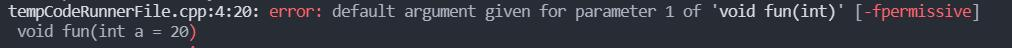#include <iostream>
using namespace std;
void fun(int);   //函数声明
int main(){
fun();
return 0;
}
void fun(int a = 20) //函数定义处不允许默认参数，应改为 void fun(int a){};
{
cout << "你好" << endl;
}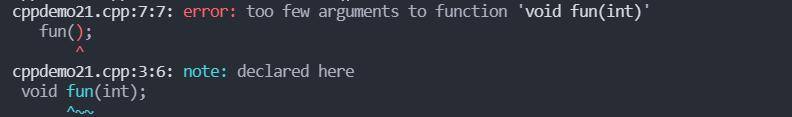2、一个函数中可以有多个默认参数，默认参数应从右至左逐渐定义，当调用函数时，从左向右匹配参数。

void foo(int a, int b = 0, bool c);    //fail,b不是最后一参数，只要有一个参数是默认参数，它后面的所有参数都必须是默认参数

3、默认值可以是全局变量、全局常量、函数。不可以是局部变量。因为默认值是在编译时确定的，必须是静态确定的。

4、默认参数不能用于细分重载函数。

void func(int,int);
void func(int=3,int=4);
//redefinition of 'void fun(int, int)'
void func(int);
void func(int,int=4);
//call of overloaded 'fun(int)' is ambiguous

5、函数的定义和声明中，都可以省略形参名

void print (int ,int);
void print(int a,int){
cout<<a<<endl;
}
void func(){
print(1,2);
}

# 指针和引用

## 指针运算符

“ * ”称为指针运算符（间接访问运算符），表示指针所指向的变量的值。一元运算符

“ & ”称为取地址运算符，用来得到一个对象的地址。一元运算符。

## void 指针与NULL指针值

（1）void

• void 指针（void *p) 空类型指针 不指向任何类型，仅仅是一个地址。
• 不能进行指针运算，也不能进行间接引用。
• 其他类型指针可以赋值给空类型指针
• 空类型指针经显示转换后方可赋给其他指针。

（2）NULL

• NULL是空指针，不指向任何地方。
• 任何类型的指针都可以赋该值。

## 指针和常量

（1）使用const 说明常量：int const x=2; 或const int x=2;

1. 需要初始化的数据成员是对象(继承时调用基类构造函数)
2. 需要初始化const修饰的类成员
3. 需要初始化引用成员数据
4. 类中含有对象成员，但其类型中，没有无参构造函数，必须在初始化列表中指明相应的有参构造函数。
5. 基类中缺少无参构造函数，必须在初始化列表中指明基类的有参构造函数。

（2）指针常量： 在指针定义语句的指针名前加const, 表示指针本身是常量。 int a; int* const p=&a; 定义时必须初始化 指针值p不可以修改，指针指向的内容可以修改。 即p是常量，不可以作为左值进行运算，*p可以修改，p不可以修改

 int *const q＝&c；    // 错误（不允许外界提供修改常量的漏洞）

（3）常量指针： 在指针的定义类型前加const，表示指向的对象是常量。 如const int *pint const *p; 均可。 以上定义表明,*p是常量，不能将*p作为左值进行操作。 定义指向常量的指针时，定义时不必须先初始化*p不可以修改，p可以修改

（4）指向常量的指针常量(常量指针常量） 形式： const int *const p＝&a； 定义时必须初始化 p与*p都是常量。他们都不能作为左值进行操作

（5）常量的特殊用法：int f(int b) const;

（6）重载和const形参

void f(int* p);
void f(const int* cp);
//有效重载，是不是指向const对象
void f(int* p );
void f(int * const pc);
//无效重载，重定义不能基于指针本身是否const 实现重载

（7）new和delete进行动态内存分配和释放

int *p；　p=new  int （100）；//动态分配一个整数并初始化
delete p;
int *p；p=new  int； //分配一个含有10个整数的整形数组　　　delete[ ] p； //删除这个数组

• new自动计算要分配类型的大小，不使用sizeof运算符，比较省事，可以避免错误。
• 自动地返回正确的指针类型，不用进行强制指针类型转换。
• 可以用new对分配的对象进行初始化。
• 不用使用头文件声明（malloc.h),更简洁。

new操作符若申请成功，返回首单元地址；否则返回NULL值。

## 函数指针和指针函数

（1）指针函数：返回指针值的函数。

int* f(int a);

（2）函数指针：指向函数地址（程序区）的指针。与函数名等价的指针。函数名是指向函数的指针常量

//类型 (*变量名) (形参表)；
//（）的优先级大于*
int f1(int n);
int (*pf1)(int n);
pf1=f1; //pf1是与f1等价的函数指针

（3）通过函数指针来调用函数：

int add(int a,int b){return a+b;}
int main()
{
int (*p)(int,int);
cout<<(*p)(3,5);   　　//四种调用形式效果等价
cout<<p(3,5);
return 0;
}
//结果：8888　

（4）函数指针作函数形参：

#include <iostream>
using namespace std;
int sum(int a, int b){
return a + b;
}
int sub(int a, int b){
return a - b;
}
int get(int (*p)(int a, int b), int m, int n){
return p(m, n);
}
int main(){
cout << "sum=" << get(sum, 3, 3) << endl;
cout << "sub=" << get(sub, 3, 3) << endl;
return 0;
}
//sum=6
//sub=0

（5）用typedef 来简化函数指针

typedef int (*FUN)(int a,int b);
int f(int,int);
FUN funp=f;
//FUN 不是指针变量，是指针类型名。
typedef  int FUNC(int,int); //先定义函数类型
FUNC* funp=f;     

#include <iostream>
using namespace std;
int sum(int a, int b){
return a + b;
}
int sub(int a, int b){
return a - b;
}
int get(int (*p)(int a, int b), int m, int n){
return p(m, n);
}
typedef int (*Func)(int a, int b);
typedef int Func2(int a, int b);
int main(){
Func func = sum;
Func2 *func2 = sub;
cout << "sum=" << get(func, 3, 3) << endl;
cout << "sub=" << get(func2, 3, 3) << endl;
return 0;
}

（6）函数的返回类型可以是函数指针

 typedef int (*SIG)  ();
typedef void (*SIGARG)  ();
SIG signal (int,SIGARG);

## 引用

（1）为一个变量、函数等对象规定一个别名，该别名称为引用。

（2）声明引用：

 int& ir=i;            //定义引用ir作为对象i的别名

（3）引用必须初始化。引用一旦被声明则不能再修改.

int  j,k;
int &s＝j;     int &s=k;  //(错误)
int & i;                  //错误
extern int&  r3           //ok，r3在别处初始化
void &a=3;//error
//void 本质不是一个类型，只是在语法上相当于一个类型，没有该类型的对象。

（4）形参和实参结合规则：

（5）引用和指针的关系

（6）用const 限定引用

int  i;   const int& p=i;

（7）引用的使用－用引用返回值

float& f2(float r){
t=3.14*r*r;
return(t);
}

（8）引用的使用－函数调用作为左值

char  a[ ]="abcdefghij";
char *q=a;
int *p=(int *)a;
while(*q)
*q++=*q+1;         //++运算符优先于*  等价于*(q++)=*q+1;  *a=bcdefghijk
p+=2;           //char的大小是1字节，int的大小是4字节，p移动了8字节printf("%s",p); //sizeof(char)=1 //sizeof(int)=4//答案是jk

# 类

## 从结构到类

C++采用“类”来支持对象，同类对象实体抽象出其共性，形成类（数据类型），类封装了数据与处理数据的过程（函数）

C++的类中既能包含数据成员，又包含函数成员或称成员函数

class class_name_identifier
{
public:         //访问控制符
公共数据成员声明语句；
公共函数成员声明语句；
private:
私有数据成员声明语句；
私有函数成员声明语句；
protected :
保护数据成员声明语句；
保护函数成员声明语句；
};    

C++中类与结构的区别： 默认情况下，class定义的成员是private的,而struct 定义的成员是public的。

## 对象的引用以及返回对象的函数

void add (Student &b){
//函数体
}
Student get(){
//函数体        　Student tmp;　return tmp
}

## 公有&保护&私有成员

 访问权限 类内成员函数或者数据成员 派生类 类外 友元函数 public yes yes yes yes protected yes yes no yes private yes no no yes

## 定义成员函数

（1）在类的内部定义成员函数

class A{
private:
int a;
public:
void show(){
cout << "haha" << endl;
}
};

::是作用域区分符、作用域运算符、名字空间引导符

#include <iostream>
using namespace std;
int month = 10;
void show(){
cout << "show" << endl;
}
class A{
public:
void show(){
::show();     //调用全局函数
}
};
int main(){
int month = 5;
cout << "局部month=" << month << endl;
cout << "全局month=" << ::month << endl;
A a;
a.show();
return 0;
}

（2）在类之后定义成员函数

class A{
private:
int a;
public:
void show();
};
void A::show(){
cout << "haha" << endl;
}

## 程序结构

（1）文件结构

1、一般情况下， c++的类定义和成员函数定义分离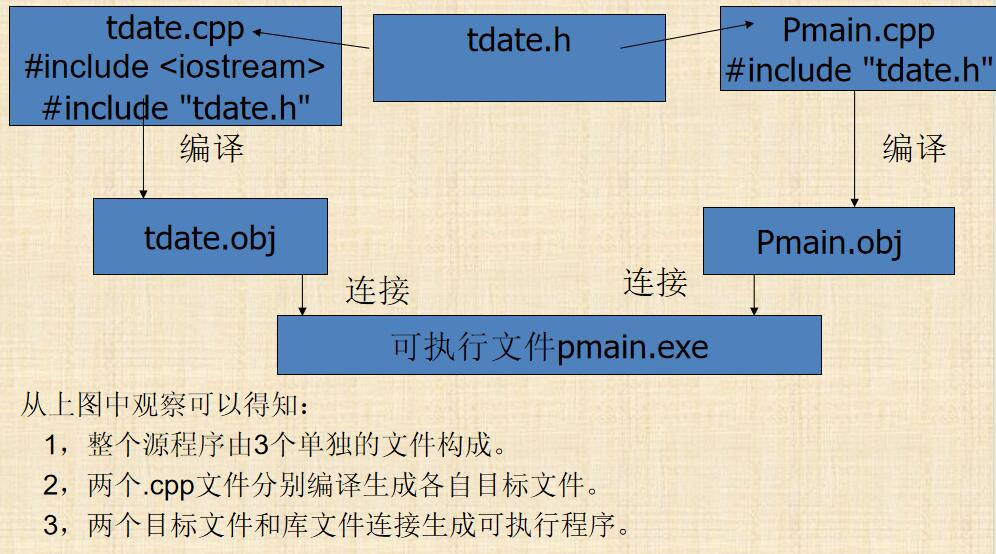< >：用于c++提供的头文件,一般存放在c++系统目录中的include 子目录下

“ ”：首先在当前文件所在的目录中进行搜索,找不到,再按标准方式进行搜索.

2、类定义和使用的分离

3、头文件卫士

Student.h文件

#ifndef STUDENT
#define STUDENT
class Student{
public:
void p(){
cout<<score;
}
float score;
protected:
char name;
int age;
};
#endif

（2）c++程序中的函数的组织方式

// a1.h  a1.cpp提供的资源
void f1();

// a2.h　 a2.cpp提供的资源
void p();

// a3.h　 a3.cpp提供的资源
void g1();
void g2();
void f2();
void h();

// s.cpp
#include”a2.h”
#include”a3.h”
void s()
{
if(…){
p();
g1();
}else{
g2();
h();
}
}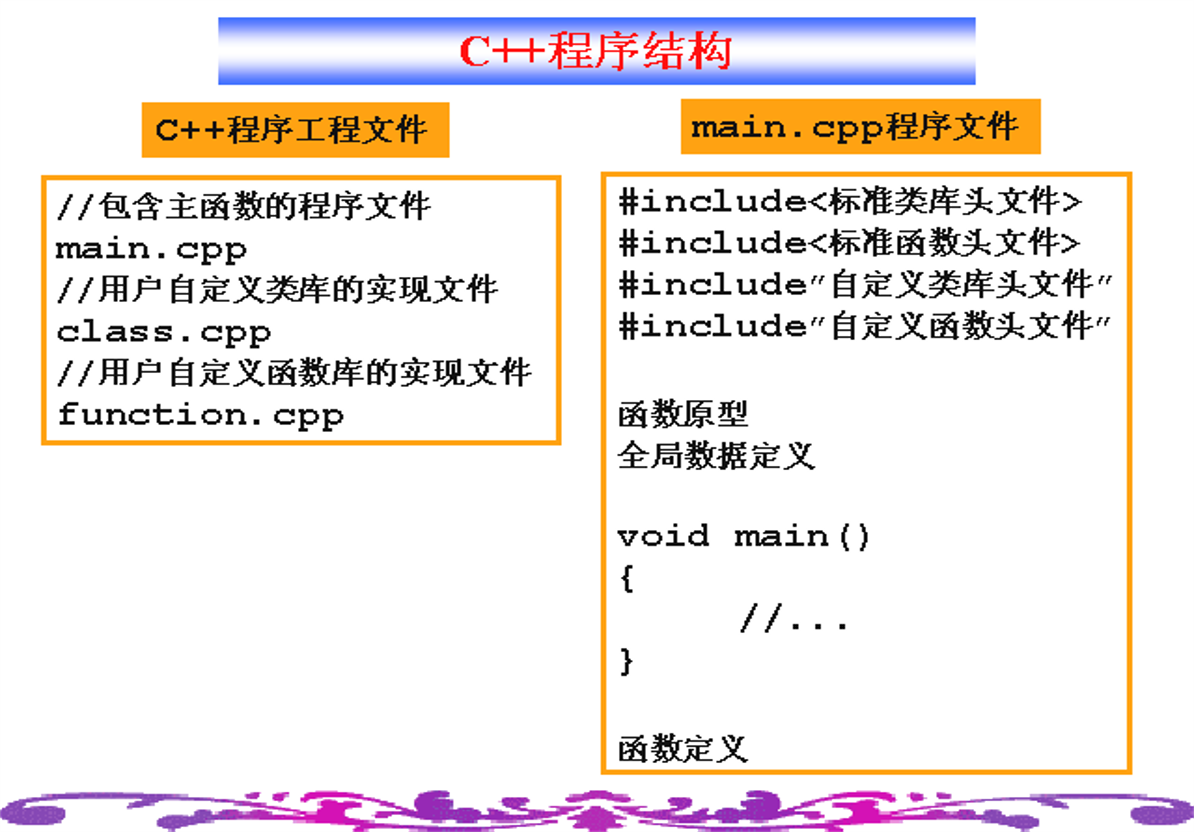## 成员函数的显式内联

class Date {
int year, month, day;
void  set ( int y, int m, int d ) {        // 默认内联
year=y; month=m; day=d;
}
bool  isLeapYear ( );
};
inline  bool  Date::isLeapYear ( )  // 显式内联
{     return ! ( year%400 ) || !(year%4) &&( year%100);
}
//类体外定义一目了然  增强可读性
//必须将类定义和成员函数的定义都放在同一个头文件或者同一个源文件中，否则编译时无法置换。

## this 指针

this是个指针常量

this是对当前对象的引用

this 指针 是一个隐含于每一个类的成员函数中的特殊指针（包括析构函数和构造函数），它用于指向正在被成员函数操作的对象。

#include <iostream>
using namespace std;
class A{
public:
int age = 0;
this->age++;
}
A &p(){
return *this;
}
};
int main(){
A a;
(a.p().age)++;
cout << a.age;
return 0;
}　　　　//1

## 类的封装

（1）保护类的内部数据不被肆意侵犯

（2）是类对它本身内部实现的维护负责，因为只有类自己才能访问该类的保护数据，所以对一切保护数据的维护只有靠类自己。

（3）限制类与外部世界的接口。 保护成员对使用者不可见。

（4）减少类与其它代码的关联程度。类的功能是独立的，不依赖于应用程序的运行环境。

## 屏蔽类的内部实现

class A{
private:
int age = 0;
public:
int getAge(){
return this->age;
}
void setAge(int age){
this->age=age;
}
};(2)类的作用域

## 作用域

(1)变量的作用域－｛｝内或其后的全部内容

(2)类的作用域

• 当内层的变量和外层的变量同名时，在内层里，外层的变量暂时地失去了可见性。
• 不能在同一作用范围内有同名变量

1）如果一个非类名隐藏了类名，则类名通过加前缀即可

class Sample
{//…..};
void fun(int Sample)
{ class Sample s;  //定义一个对象要加前缀
Sample++;
//…}

2）如果一个类名隐藏了非类名，则用一般作用域规则即可

int s=0;
void func()
{ class s{//…};
s a:
::s=3;}  // class作用域到此结束
int g=s; //在函数中定义的类称为局部类，局部类的成员函数必须在类定义内部定义

## 名字空间

#include <iostream>
using namespace std;
namespace name
{
int a = 20;
void show()
{
cout << "I'm a namespace" << endl;
}
namespace another
{
int b = 30;
}
}
int main()
{
cout << name::a << endl;
name::show();
cout << name::another::b << endl;
return 0;
}//20//I'm a namespace//30

using namespace std;
//在std中定义和声明所有标识符都可以作为全局量来使用。

OOA：面向对象分析(干什么)

OOD：面向对象设计（怎样干）

OOP：面向对象编程（实现）

# 构造、析构函数

## 构造、析构函数

1. 构造函数是特殊的类成员函数。
2. C++规定与类同名的成员函数是构造函数，在该类的对象创建时，自动被调用
3. 构造函数负责对象的初始化 可拥有多个参数。
4. 可以重载
5. 构造函数不返回具体的值，不指定函数的返回类型。
6. 可内置定义，也可以在类外定义。
7. 通常是Public

1. 析构函数也是一个特殊的成员函数；
2. 作用与构造函数相反；
3. 在对象的生命期结束时自动被调用
4. 名字是类名前加一个~
5. 不返回任何值；没有函数类型，没有函数参数，因此不能被重载
6. 一个类可以由多个构造函数，只能有一个析构函数
7. 可内置定义，也可以在类外定义。
8. 用于清理和释放资源的工作。
9. 通常是Public的
10. 析构函数并不是销毁对象的，只是释放构造函数在构造时初始化的资源（主要包括堆上分配内存等）
#include <iostream>
using namespace std;
class A{
private:
int a;

public:
A(){
cout << "Default constructor called" << endl;
}
A(int a){
this->a = a;
cout << "Constructor called" << endl;
}
~A(){
cout << "Destructor called" << endl;
}
};
int main(){
A a;
A b(1);
return 0;
}

//Default constructor called
//Constructor called
//Destructor called
//Destructor called

## 组合类

#include <iostream>
using namespace std;
class Student{
public:
Student(){
cout << "Student constructor called" << endl;
}
~Student(){
cout << "Student destructor called" << endl;
}
};
class Teacher{
public:
Teacher(){
cout << "Teacher construtor called" << endl;
}
~Teacher(){
cout << "Teacher destrctor called" << endl;
}
};
class Manager{
private:
Student student;
Teacher teacher;
public:
Manager(){
cout << "Manager constructor called" << endl;
}
~Manager(){
cout << "Manager destructor called" << endl;
}
};
int main(){
Manager manager;
return 0;
}

//Student constructor called
//Teacher construtor called
//Manager constructor called
//Manager destructor called
//Teacher destrctor called
//Student destructor called

//注意执行顺序，组合类的对象成员先初始化，调用构造函数，然后是本类初始化，调用构造函数，析构顺序相反
#include <iostream>
using namespace std;
class Student{
public:
Student(){
cout << "Student constructor called" << endl;
}
~Student(){
cout << "Student destructor called" << endl;
}
};
class Teacher{
public:
Teacher(){
cout << "Teacher construtor called" << endl;
}
~Teacher(){
cout << "Teacher destrctor called" << endl;
}
};
class Temp{
public:
Temp(){
cout << "Temp constructor called" << endl;
}
~Temp(){
cout << "Temp destructor called" << endl;
}
};
class Manager : public Temp{
private:
Student student;
Teacher teacher;
public:
Manager(){
cout << "Manager constructor called" << endl;
}
~Manager(){
cout << "Manager destructor called" << endl;
}
};
int main(){
Manager manager;
return 0;
}

//Temp constructor called
//Student constructor called
//Teacher construtor called
//Manager constructor called
//Manager destructor called
//Teacher destrctor called
//Student destructor called
//Temp destructor called

#include <iostream>
using namespace std;
class Student{
public:
Student(){
cout << "Student constructor called" << endl;
}
~Student(){
cout << "Student destructor called" << endl;
}
};
class Teacher{
public:
Teacher(){
cout << "Teacher construtor called" << endl;
}
~Teacher(){
cout << "Teacher destrctor called" << endl;
}
};
class Temp
{
public:
Temp(){
cout << "Temp constructor called" << endl;
}
~Temp(){
cout << "Temp destructor called" << endl;
}
};
class Temp2{
public:
Temp2(){
cout << "Temp2 constructor called" << endl;
}
~Temp2(){
cout << "Temp2 destructor called" << endl;
}
};
class Manager{
private:
Student student;
Teacher teacher;
public:
Manager(){
Temp temp;               //局部对象Temp
Temp2 temp2;             //局部对象Temp2
cout << "Manager constructor called" << endl;
}
~Manager(){
cout << "Manager destructor called" << endl;
}
};
int main(){
Manager manager;
return 0;
}

/*
Student constructor called
Teacher construtor called
Temp constructor called
Temp2 constructor called
Manager constructor called
Temp2 destructor called
Temp destructor called
Manager destructor called
Teacher destrctor called
Student destructor called
*/

#include <iostream>
using namespace std;
class A
{
private:
const int a;      //常数据成员
int &b;           //引用

public:
A(int a, int b) : a(a), b(b)
{
cout << "A constructor called" << endl;
}　/*A(int a,int b){    //错误　　　　this->a=a;　　　　this->b=b;　}*/
};
int main()
{
A a(10, 20);
return 0;
}

## 常对象和常成员函数

#include <iostreamusing namespace std;
class A{
private:
const int a;
int &b;

public:
A(int a, int b) : a(a), b(b){}
void show() const; //构成重载
void show()        //如果不是常对象，则优先调用普通函数
{
cout << "normal show" << endl;
}
};
void A::show() const //类外实现常成员函数时不可省略const
{
cout << "const show" << endl;
}
int main(){
A a(10, 20);
a.show();         //normal show
const A b(10,20);
b.show();         //const show
return 0;
}

## 为对象申请动态空间

int *p=new int;
delete p;
int *q=new int;
delete []q;

## 拷贝构造函数

类名 (类名& 形参); 或者是 类名 ( const 类名& 形参);

Student (Student& s);或者是Student ( const Student& s);

#include <iostream>
using namespace std;
class B{
private:
int a;
public:
B(const B &b) {}
};
int main(){
B b;
return 0;
}
//no matching function for call to 'B::B()'

class aa {
public:
aa(){f=new char;}
aa(aa const & s){
f=new   char;    //开辟空间　　strcpy(f,s.f);}       //数据复制
~aa(){delete [ ]f;}
char * f;
};
int main()
{aa p;
strcpy(p.f,"Computer");
cout<<p.f<<endl;
aa q(p);
cout<<q.f<<endl;
strcpy(p.f,”Software”);
cout<<p.f<<q.f<<endl;
return 0;}

#include <iostream>
using namespace std;
class Data
{
public:
Data(int x = 0)
{
Data::x = x;
cout << "Data()" << endl;
}
Data(const Data &)
{
cout << "Data(const Data&)" << endl;
}
~Data()
{
cout << "~Data()" << endl;
}

private:
int x;
};
class Base
{
public:
Base()
{
cout << "Base()" << endl;
}
Base(const Base &)
{
cout << "Base(const Base&)" << endl;
}
virtual ~Base() {}
};
class Child : public Base
{
public:
Child()
{
cout << "Child()" << endl;
}
virtual ~Child()
{
cout << "~Child()" << endl;
}

private:
Data d2;
};

int main()
{
Child c1;
Child c2(c1);
return 0;
}
/*
Base()
Data()
Child()
Base(const Base&)
Data(const Data&)
~Child()
~Data()
~Child()
~Data()
*/

## 关于无名对象的构造与使用：

　Student(9);// 无名对象 ，正确
Student *p=&Student(8);//错误，error: taking address of temporary
Student const &q=Student(7);//正确，无名对象将在它的引用离开作用域时销毁
Student i(6);
i = Student(5);//正确　Student &q=Student(5);  //错误cannot bind non-const lvalue reference of type 'Student&' to an rvalue of type 'Student'

## 构造函数用于类型转换

class aa {
public:
aa(int a=1){id=a;cout<<“构造”<<endl;}
aa (aa & s){id = s.id;cout<<“拷贝”;}
int & Getid(){return(id);}
private:
int id;
};
int main()
{ aa m;         //“构造”
aa n(m);     //“拷贝”
aa o = m;    //“拷贝”  和aa o(m)等价
aa s = 9;    //“构造”  和aa s(9)等价
aa t;        //“构造”
t = 9;       // t=aa(9);“构造”、  赋值运算
return 0;
}               //隐式的类型转换，可以将其他类型转换成类类型

class aa {
public:
aa(int a=1){id=a;}
int id;
};
class bb {
public:
bb(int a=2){id=a;}
int id;
};
void m(aa a)
{cout<<a.id<<endl;}
void m(bb a)
{cout<<a.id<<endl;}
int main()
{   m(9);  //存在二义性;  m(bb(9));
return 0;
}//转换不能太复杂，不允许多参数，不允许间接转换

explicit可以禁止隐式类型转换

explicit A(int a){this->a=a}
A a=3;   //错误error: conversion from 'int' to non-scalar type 'A' requested
A a(3);   //正确

## 友元

（1）声明的位置既可在public区，也可在protected区。友元函数虽然是在类内进行声明，但它不是该类的成员函数，不属于任何类

（2）在类外定义友元函数，与普通函数的定义一样，一般与类的成员函数放在一起，以便类重用时，一起提供其友元函数。

（3）友元函数是能访问类的所有成员的普通函数，一个函数可以是多个类的友元函数，只需在各个类中分别声明。

（4）友元能使程序精炼，提高程序的效率。

（5）友元破坏了类的封装，使用时，要权衡利弊,在数据共享与信息隐藏之间选择一个平衡点。

（6）友元类的所有成员函数都可视为该类的友元函数，能存取该类的私有成员和保护成员。

（7）友元关系不具有对称性。 友元关系不具有传递性。如果类B是类A的友元类，类C是类B的友元类，这并不隐含类C是类A的友元类

#include <iostream>
#include <string>
using namespace std;
class Building;
//友元类
class MyFriend
{
public:
//友元成员函数
void LookAtBedRoom(Building &building);
void PlayInBedRoom(Building &building);
};
class Building
{
//全局函数做友元函数
friend void CleanBedRoom(Building &building);
#if 1
//成员函数做友元函数
friend void MyFriend::LookAtBedRoom(Building &building);
friend void MyFriend::PlayInBedRoom(Building &building);
#else
//友元类
friend class MyFriend;
#endif
public:
Building();

public:
string mSittingRoom;

private:
string mBedroom;
};

void MyFriend::LookAtBedRoom(Building &building)
{
cout << "我的朋友参观" << building.mBedroom << endl;
}
void MyFriend::PlayInBedRoom(Building &building)
{
cout << "我的朋友玩耍在" << building.mBedroom << endl;
}

//友元全局函数
void CleanBedRoom(Building &building)
{
cout << "友元全局函数访问" << building.mBedroom << endl;
}

Building::Building()
{
this->mSittingRoom = "客厅";
this->mBedroom = "卧室";
}

int main()
{

Building building;
MyFriend myfriend;

CleanBedRoom(building);
myfriend.LookAtBedRoom(building);
myfriend.PlayInBedRoom(building);

system("pause");
return EXIT_SUCCESS;
}
/*

*/

## 静态成员

int StudentID::ID=0;

(1) 不管一个类的对象有多少个，其静态数据成员也只有一个，由这些对象所共享，可被任何一个对象所访问。

(2) 在一个类的对象空间内，不包含静态成员的空间，所以静态成员所占空间不会随着对象的产生而分配，或随着对象的消失而回收。

(3) 静态数据成员的存储空间的分配是在程序一开始运行时就被分配。并不是在程序运行过程中在某一函数内分配空间和初始化。

(4) 静态数据成员的初始化语句，既不属于任何类，也不属于包括主函数在内的任何函数，静态数据成员初始化语句最好在类的实现部分定义

(5) 对于在类的public部分说明的静态数据成员，可以不使用成员函数而直接访问，既使未定义类的对象，同样也可以直接访问，但在使用时也必须用类名指明所属的类。 而private和protected部分的静态成员只能通过类的成员函数访问。

(6) 不允许不指明对象访问非静态数据成员；不允许使用this

(7)可以用const限定静态成员吗？

class A{
public:
const static int a;
static void getA() const{  //编译报错
return a;
}
};
//静态成员函数上不允许使用类型限定符
class A{
private:
int t = 10;

public:
const static int a;
static int getT(){
return t;
}
};
//error: invalid use of member 'A::t' in static member function非静态成员引用必须与特定对象相对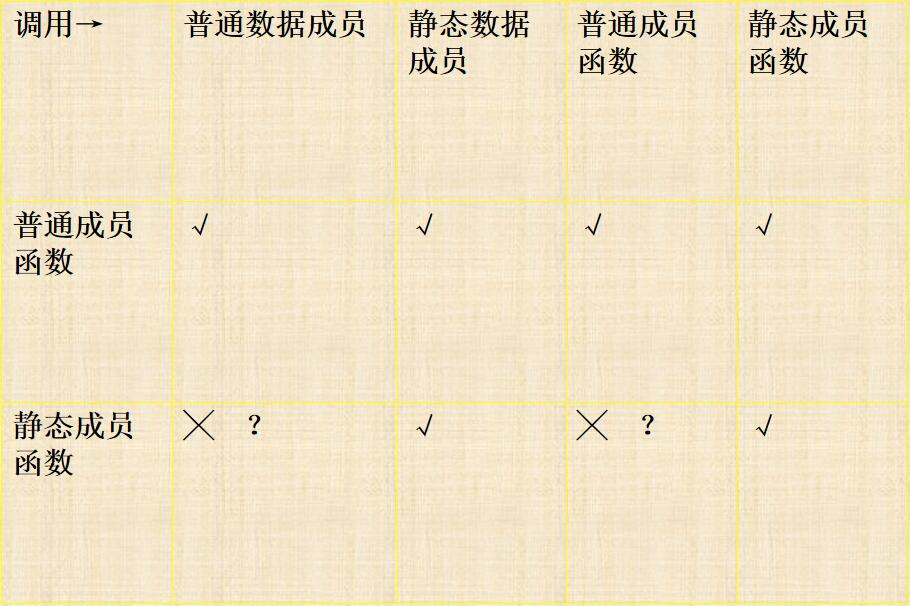## 单例模式

#include <iostream>
using namespace std;
class A{
private:
A() {}                      //构造函数私有化
A(const A &a) {}
static A *instance;
public:
void show(){
cout << "hello" << endl;
}
static A *getInstance(){
if (instance == NULL){
instance = new A;
}
return instance;
}
};
A *A::instance = NULL;         //静态数据成员类外初始化
int main(){
A *instance = A::getInstance();
instance->show();
return 0;
}
//如果写A a会报错

# 运算符重载

## 格式

(1)在C++中几乎所有的运算符（ 除“.”（成员选择符）、“.*”(成员对象选择符）、“->*（成员指针选择符）”、“::”、“？：”、“size of”外）都可以被重载。 只能重载　　　　     C++中已有的运算符,不允许创建新的运算符.

(2) 运算符的重载既不会改变原运算符的优先级和结合性。

(3) 至少有一个操作对象是自定义类型，参数都是基本类型时不能重载.

(4) 不提倡改变参数个数、运算符含义。

(5) 重载运算符的函数不能有默认的参数。

(6) 运算符重载时参数个数不可以超过原来数目

## 赋值运算符重载

void fn(A a) {
A na=a; //拷贝构造函数：将一个对象拷贝到另一个新对象
A b; 　　 b=a;
}          //赋值运算符：将一个对象为另一个已经存在的对象赋值

#include <iostream>
using namespace std;

class A
{
public:
int num;
A(int n) : num(n) {}
};
int main()
{
A a(3);
A b(0);
b.operator=(a);
cout << b.num;
return 0;
}
//3

## ++/--运算符重载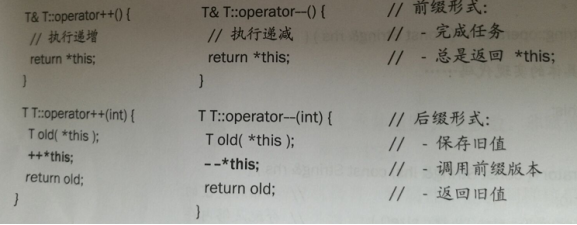## 类型转换运算符

operator 类型名（）；

#include <iostream>
using namespace std;
class aa{
float a;
float b;
public:
float &aaa() { return (a); }
float &bbb() { return (b); }
operator float();
};
aa::operator float() { return (a); }
int main(){
aa a, b;
a.aaa() = 1;
a.bbb() = 2;
b.aaa() = 3;
b.bbb() = 4;              //int(3.5)
cout << float(a) << endl; //a.operator float();
cout << 10 + a << endl;
cout << a + b << endl;
cout << a << endl;
}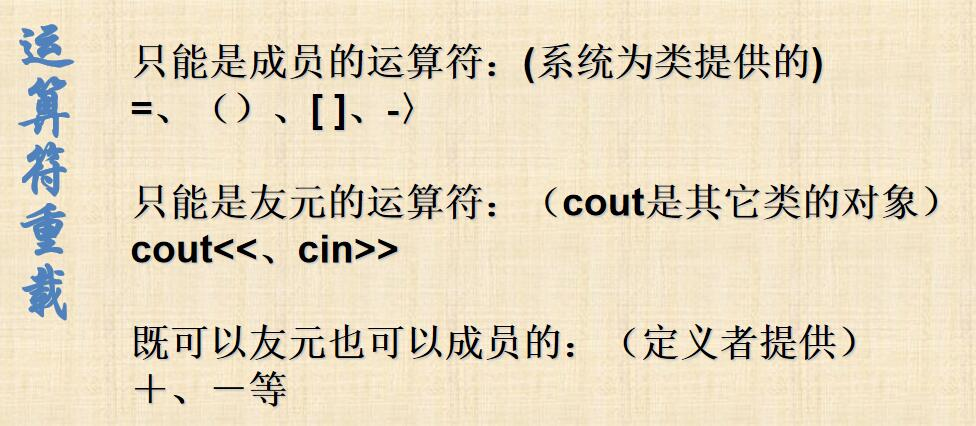## 类和友元

class Person{
friend ostream& operator<<(ostream& os, Person& person);
public:
Person(int id,int age){
mID = id;
mAge = age;
}
private:
int mID;
int mAge;
};

ostream& operator<<(ostream& os, Person& person){
os << "ID:" << person.mID << " Age:" << person.mAge;
return os;
}
//全局函数友元
class Person
{
public:
Person(int id, int age)
{
mID = id;
mAge = age;
}
ostream &operator<<(ostream &os)
{
os << "ID:" << mID << " Age:" << mAge;
return os;
}
private:
int mID;
int mAge;
};
//另一种：在类内重载<<，不需友元

# 继承

class 派生类名：继承方式  基类名
{ 派生类中的新成员 }

c++提供了类的继承机制，解决了软件的复用问题。

protected继承和private继承得到的类都不是子类 “凡是父类对象可以出现的地方可以用子类对象代替”，不再适用

#include <iostream>
using namespace std;
class Parent{};
class Child : protected Parent{};
int main(){
Child child;
Parent &parent = child;
return 0;
}
//error: 'Parent' is an inaccessible base of 'Child'
//不允许对不可访问的基类 "Parent" 进行转换

#include <iostream>
using namespace std;
class Parent{
private:
int a;
public:
Parent(int a){
this->a = a;
}
};
class Child : protected Parent{
public:
Child(int a) : Parent(a) //正确
{
//Parent(a)错误no matching function for call to 'Parent::Parent()
}
};
int main(){
return 0;
}

（1）基类构造函数，

（2）派生类对象成员类的构造函数（如果有的话）。

（3）派生类构造函数体中的内容。

## 嵌套类

class A{
int a;
public:
class B{
int b;
public:
B(int i);
};
void f(){ pb->b=5; //error}
B* pb;
};
A::B::B(int i):b(i){}  //成员可以在体外定义
int main(){
A a;
A::B b(10);
}

## 多重继承和虚基类

class M_p:public Master,public Phd{}

(1)    所有基类的构造函数；多个基类构造函数的执行顺序取决于定义派生类时所指定的顺序，与派生类构造函数中所定义的成员初始化列表的参数顺序无关。

(2)    对象成员（如果有的话）的构造函数；

(3)    派生类本身构造函数的函数代码。

class 派生类名：virtual public 基类名 { //声明派生类成员 }；

C++规定，虚基类子对象是由最后派生类的构造函数通过调用虚基类的构造函数进行初始化的。

（1）任何虚拟基类的构造函数按照他们被继承的顺序构造

（2）任何非虚拟基类的构造函数按照他们被继承的顺序构造

（3）任何成员对象的构造函数按照他们被声明的顺序构造

（4）类自己的构造函数

（1）如果不用虚继承，用多继承：

#include <iostream>
using namespace std;
class Grandfather
{
public:
Grandfather()
{
cout << "Grandfather constructor called" << endl;
}

protected:
int a = 0;
void fun() {}
};
class FatherA : public Grandfather
{
public:
FatherA()
{
cout << "FatherA constructor called" << endl;
}
void show()
{
cout << "FatherA" << endl;
}
};
class FatherB : public Grandfather
{
public:
FatherB()
{
cout << "FatherB constructor called" << endl;
}
void show()
{
cout << "FatherB" << endl;
}
};
class Son : public FatherA, public FatherB
{
public:
Son()
{
cout << "Son constructor called" << endl;
}
void show()
{
cout << "Son" << endl;
}
};
int main()
{
// Grandfather grandfather;
// FatherA fatherA;
// FatherB fatherB;
Son son;
cout << "sizeof(Son)=" << sizeof(son) << endl;
// cout << "sizeof(Grandfather)=" << sizeof(grandfather) << endl;
// cout << "sizeof(fatherA)=" << sizeof(fatherA) << endl;
// cout << "sizeof(fatherB)=" << sizeof(fatherB) << endl;
return 0;
}
/*
Grandfather constructor called      //间接基类构造函数调用了两次
FatherA constructor called
Grandfather constructor called
FatherB constructor called
Son constructor called
sizeof(Son)=8                       //Son类中继承了两个间接基类的数据成员
*/

（2）使用虚继承#include <iostream>using namespace std;

class Grandfather
{
public:
Grandfather()
{
cout << "Grandfather constructor called" << endl;
}

protected:
int a = 0;
void fun() {}
};
class FatherA : virtual public Grandfather
{
public:
FatherA()
{
cout << "FatherA constructor called" << endl;
}
void show()
{
cout << "FatherA" << endl;
}
};
class FatherB : virtual public Grandfather           //虚继承
{
public:
FatherB()
{
cout << "FatherB constructor called" << endl;
}
void show()
{
cout << "FatherB" << endl;
}
};
class Son : public FatherA, public FatherB            //虚继承
{
public:
Son()
{
cout << "Son constructor called" << endl;
}
void show()
{
cout << "Son" << endl;
}
};
int main()
{
// Grandfather grandfather;
// FatherA fatherA;
// FatherB fatherB;
Son son;
cout << "sizeof(Son)=" << sizeof(son) << endl;
// cout << "sizeof(Grandfather)=" << sizeof(grandfather) << endl;
// cout << "sizeof(fatherA)=" << sizeof(fatherA) << endl;
// cout << "sizeof(fatherB)=" << sizeof(fatherB) << endl;
return 0;
}
/*
Grandfather constructor called                        //间接基类构造函数只调用了一次
FatherA constructor called
FatherB constructor called
Son constructor called
sizeof(Son)=24 //注意这里Song的内存大小，（间接基类的int num占4,两个直接基类的两个虚函数表占8+8=16，然后根据字节对齐规则，总内存20扩大到8的倍数也就是24
*/

# 多态

## 虚函数

（1）在基类用virtual声明成员函数为虚函数

（2）在派生类中重新定义此函数，要求函数名函数的参数个数类型全部与基类的虚函数相同，并根据派生类的需要重新定义函数体

（3）定义一个指向基类对象的指针变量，并使它指向同一类族中的某一对象。

（4）通过该指针变量调用此虚函数，此时调用的就是指针变量指向的对象的同名函数。 c++规定，当一个成员函数被声明为虚函数后，其派生类中原型相同的函数都自动成为虚函数 。派生类没有对基类的虚函数重新定义，则派生类继承其直接基类的虚函数。

static_cast:静态转型，必须是相关类型，非多态类层次的祖孙互易，void*转换任何类型

dynamic_cast：动态转型，专门针对有虚函数的继承结构，将基类指针或引用转换成想要的子类指针或引用

const_cast：常量转型，去掉常量性的转换。

dynamic_cast<Derived*>(p);

static_cast<Car*>(p);

const char* max(const char*,const char*);

char* p=const_cast<char*>(max(“hello”,”good”));

Virtual出现则每个类增加一个虚函数表保存类的虚函数 。凡有虚函数的类均维护一个虚函数表 ，实例化每个对象中会增加一个指针指向虚函数表(对象大小会有变化).。虚函数调用时不需要确定对象类型，通过该指针即可找到所要链接函数

class Student{
int move_tel;
public:   int id;
virtual Student * n(){
return(new Student(*this));}
};
class Master :public Student{
string topic;
public:  //方法略
Student * n(){
return(new Master(*this));}
};
class Phd:public Student{
string professor;
public:  //方法略
Student * n(){
return(new Phd(*this));  } };
class DoubleMaster :public Student{
string SecondMajor;
public: //方法略
Student * n(){
return(new DoubleMaster (*this));}
};
class Member{
Student * m;
public:
Member( ){ }
Member(Member &s){
for(int i=0;i<128;i++)
this->m[i] = s.m[i]->n();}
Student * &f(int k)
{return(m[k]);}
};
void main()
{Member s;
s.f(0)=new Student;
s.f(1)=new Master;
s.f(2)=new Phd;
s.f(3)=new DoubleMaster;
s.f(4)=new Phd;
Member t(s);
}
//定义了一个t，并完全拷贝s内容

## 抽象类

virtual 函数原型=0；

（1）抽象类中可以有多个纯虚函数。

（2）抽象类也可以定义其他非纯虚函数

（3）从抽象类可以派生出具体或抽象类，但不能从具体类派生出抽象类

【答】虽然抽象类不能实例化，但是抽象类被继承之后，它的派生类可以实例化；而派生类在实例化调用构造函数的时候会先调用基类中的构造函数，所以抽象类的构造函数也是可以被调用的，所以抽象类中可以有构造函数。但是注意：C++核心准则C.126:抽象类通常不需要构造函数‍，因为抽象类通常不包含任何需要构造函数初始化的数据。

## 类的六种关系

1、继承（is-a） 虎是一种动物

2、实现接口（is like a）（接口：是对行为的抽象） 飞机和鸟都会飞

1、合成、组合（is a part of）（强拥有，严格的部分整体） 鸟和翅膀；生命周期同步。 形式：成员

2、聚合（own a）（弱拥有，A可以包含B，但B不是A的一部分） 大雁和雁群，群体和个体，生命周期不同步 形式：成员，一般为容器

3、关联（has a） 人有朋友，不是包含关系。企鹅和气候的关系 形式：成员

4、依赖（use a）（运行期关系） 动物需要呼吸氧气 形式：局部变量、方法的参数或者对静态方法的调用

public class Person {
private Hand hand;

public Person() {
hand=new Hand();
}

private void go(){
hand.hand();
}

public static void main(String[] args) {
new Person().go();    //person消亡时，hand也消亡
}
}
class Hand{
public void hand(){
System.out.print("hand");
}
}

public class Person2 {
private Computer c;
public Person2(){

}
public Person2(Computer c){
this.c=c;
}

public void go(){
c.computer();
}

public static void main(String[] args) {
Computer computer=new Computer();
Person2 person = new Person2(computer);
person.go();   //person消亡时，不影响computer
}
}
class Computer{
public void computer(){
System.out.print("computer");
}
}

“高内聚低耦合”

1、单一职责原则

2、开放封闭原则

3、依赖倒转原则

4、里氏替换原则

5、合成聚合复用原则

6、迪米特法则（最少知识原则）

7、接口隔离原则

## 终结类

#include <iostream>
using namespace std;
class AA{
private:
AA() {}
public:
static AA *GetAAObj() { return new AA; }
//static AA GetAAObj(){    return AA();}
static void DeleteAAObj(AA *pa) { delete pa; }
void show(){
cout << "hehe" << endl;
}
protected:
int _aa;
};
class BB : public AA{
};
int main(){
AA::GetAAObj()->show();
//BB b;错误：无法引用 "BB" 的默认构造函数 -- 它是已删除的函数
//note: 'BB::BB()' is implicitly deleted because the default definition would be ill-formed:
// error: 'AA::AA()' is private within this context
return 0;
}

（1）典型方法

#include <iostream>
using namespace std;
class FinalParent{
protected:
FinalParent() {}
};
class FinalClass :private virtual FinalParent{
public:
FinalClass() {}
//其他略，类要实现的功能或者定义的数据成员可以写在这里
};
class A :public FinalClass{};
int main(){
FinalClass f;
A a; //错
return 0;
}
//[Note] 'A::A()' is implicitly deleted because the default definition would be ill-formed:
//[Error] 'FinalParent::FinalParent()' is protected

（2）

#include <iostream>
using namespace std;
class Vb {private:
Vb() {}                     //构造函数私有
public:
friend class Student;       //Student为友元类，可以调用基类构造函数
void show(){
cout<<"hello"<<endl;
}
};
class Student :virtual public Vb {
public:
Student(){}
};
class A :public Student {};     //A无法调用间接基类的构造函数
int main() {
Student s;
s.show();
A a;       //错
return 0;
}

## final和override关键字

1、类被final修饰，不能被继承

class A1 final { };
class B1 : A1 { }; // “B1”: 无法从“A1”继承，因为它已被声明为“final”

2、虚函数被final修饰，不能被override

class A1{
virtual void func() final {} };
class B1 : A1{
virtual void func() {}
//“A1::func”: 声明为“final”的函数无法被“B1::func”重写
};

3、被override修饰后如果父类无对应的虚函数则报错。override就是编译器辅助你检查是否继承了想要虚继承的函数

struct A1{    virtual void func(int) {}};
struct B1 : A1{    virtual void func(int) override {} // OK
virtual void func(double) override {} //错
// “B1::func”: 包含重写说明符“override”的方法没有重写任何基类方法
};

# 模板

template < 模板参数表 >

class 类型参数名 (或 typename 类型参数名)

template <class T>      //class可以用typename代替
void swap1(T& a,T& b){
T temp=a;
a=b;
b=temp;
}//函数模板
swap1<int>(a,b);//显式
swap1(a,b);//隐式

C++ 编译器在遇到调用用模板方式定义的函数时，会根据调用函数的参数类型构造出一个个具体的函数。这个过程称为函数模板的实例化（instantiation）。

template<typename T,typename U>  //模板类型声明不能共享；typename不能节省template<classname T,U> 错误
{  cout<<a+b<<endl;}
void main(){
int x=1,y=2;
float s=3.0,t=4.0;
//可以相同也可以不相同
}

#include <iostream>
using namespace std;
template <class T>
void swap(T &a, T &b){
T tmp = a;
a = b;
b = tmp;
cout << "template function called" << endl;
}
void swap(int &a, int &b){
int tmp = a;
a = b;
b = tmp;
cout << "non-template function called" << endl;
}
int main(){
int a = 1, b = 2;
swap(a, b);
cout << "a=" << a << " b=" << b;
return 0;
}
//non-template function called
//a=2 b=1

## 模板的参数是非类型参数：

template <class T, int i>
int find(T a[],T k)
{
for(int j=0;j<i;j++)
if(a[j]==k)
return(j);
return(-1);
}
void main(){
int a={0};
cout<<find<int,10>(a,1);
}

## 类 模板

template <typename T=int>//类模板也允许默认参数
class Stack{
public:
void push( T );
T pop( );
private:
};
//与普通类一样，类模板的成员函数也可以在类模板定义中定义具体实现，这时，该函数是内联的（inline）。

template <typename T,typename U> //多个类型参数
class List{… …};
List <NODE,Student>  k;

# I/O流

C++语言系统为实现数据的输入和输出定义了一个庞大的类库，它包括的类主要有:

ios：抽象基类

iostream：输入流类istream，输出流类ostream，输入输出流类iostream;

对标准输入设备和标准输出设备的输入输出，简称为标准I/O流

fstream：输入文件流类ifstream，输出文件流类ofstream，输入输出文件流类fstream;

对在外存磁盘上文件的输入输出，简称为文件I/O流

Strstream:输入字符串流类istrstream，输出字符串流类ostrstream，输入输出字符串流类strstream.

sstream:输入字符串流类istringstream，输出字符串流类ostringstream，输入输出字符串流类stringstream.

对内存中指定的空间进行输入输出。通常指定字符数组string类对象做为存储空间的输入输出，简称为串I/O流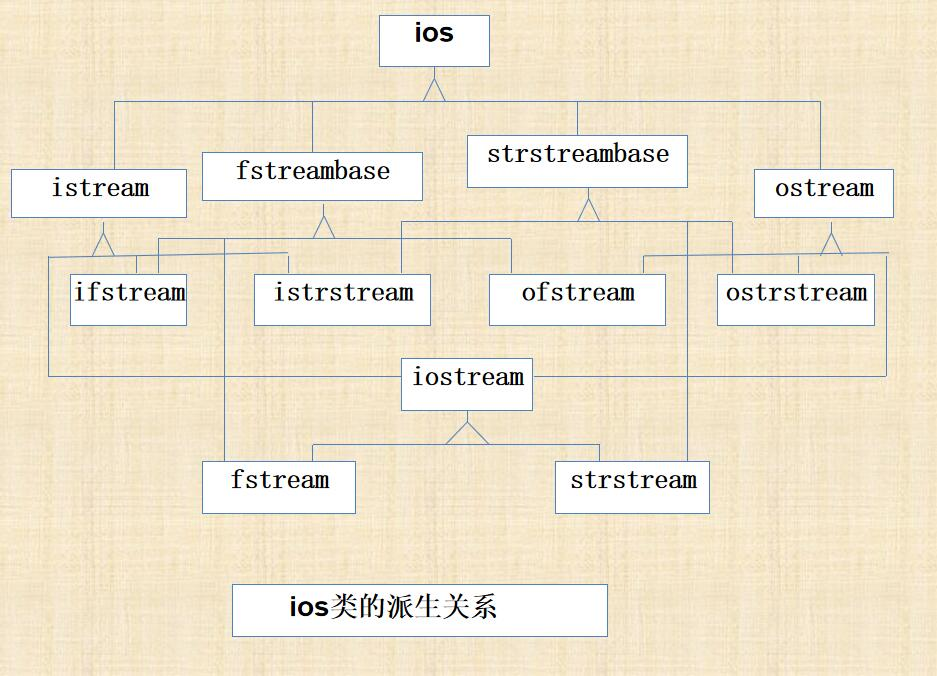# 异常处理

（1）框定异常（try语句块） 将那些有可能产生错误的语句放在try块中

（2）抛掷异常（throw语句） 检测是否产生异常，若是，则抛掷异常。

（3）捕捉异常,定义异常处理（catch语句块）将异常处理语句放在catch块中,以便异常被传递过来时就处理它。

C++只理会受监控的异常。 Try块之后必须紧跟一个或多个catch语句。 Catch括号中只能容纳一个形参，与throw抛掷的异常类型匹配时，便捕获了异常。 避免把正常逻辑淹没在错误处理代码中，从而使程序更易于阅读。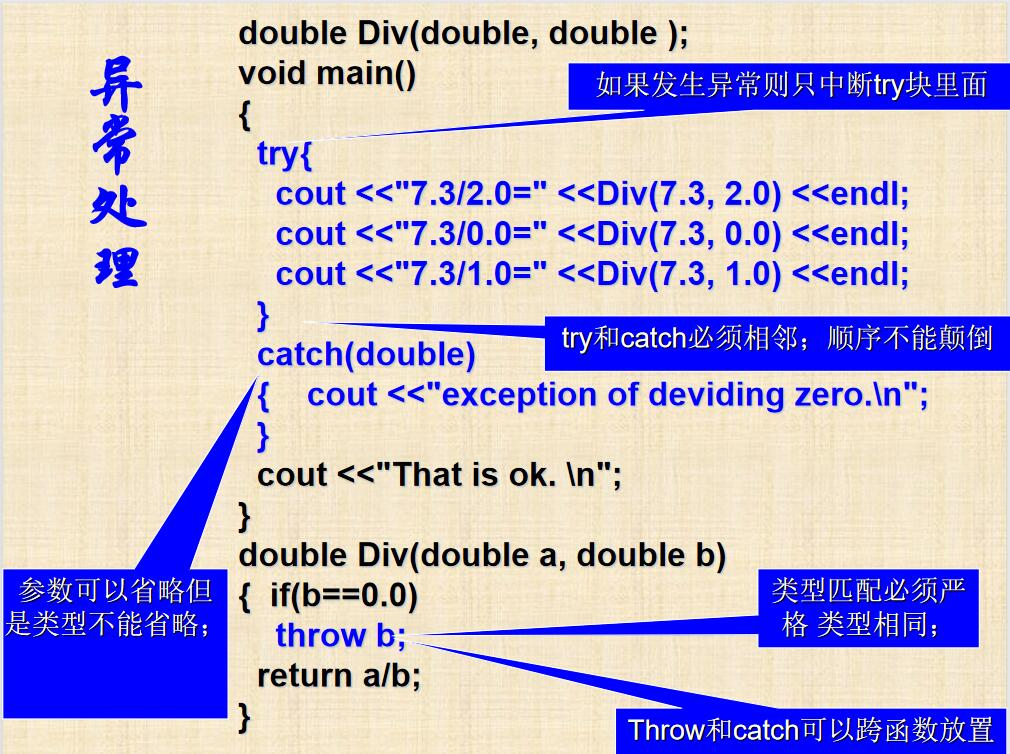class A{
char net;
public:
virtual  void x(){cout<<"网络错误";}
};
class B:public A{
long card;
public:
void x(){cout<<"网卡错误";}
};
class C:public B{
int port;
public:
void x(){cout<<"端口错误";}
};
void net()         //void net() throw(A,B,C)
{  throw B();}
--------------------------------------------------
void net() throw(A,B,C);  //异常申述(异常说明) 声明和定义要一致
void main()
{      try{net();}
catch(A & s){s.x();}   }

posted @ 2021-06-25 20:57  gonghr  阅读(1440)  评论(0编辑  收藏  举报